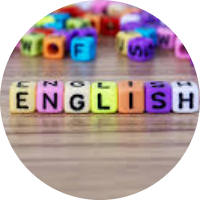# Sets: Fundamentals and study

These MCQs help students to learn about the concept of the set which serves as a fundamental part of present-day mathematics. Today this concept is being used in almost every branch of mathematics. Sets are used to define the concepts of relations and functions. The study of geometry, sequences, probability, etc. requires the knowledge of sets.

Start Quiz

Which of the following set is not possible?

Honest persons Prime numbers up to 100 Even numbers up to 100 Letters forming the word SCHOOL

Write solution set of equation x2-3x+2=0 in roster form.

{1,3} {2,4} {1,4} {1,2}

Write the set {x : x is an integer and x2-9=0} in roster form.

{3} {-3} {3,-3} {9,3}

If the number of elements in a set S are 5. Then the number of elements of the power set P(S) are?

5 6 16 32

If x ≠ 1, and f(x) = x + 1 / x – 1 is a real function, then f(f(f(2))) is

2 1 4 3

X < 0, Y < 0 X > 0, Y < 0 X < 0, Y > 0 X < 0, Y > 0

{ (A, B) : A² +B² = 1} on the sets has the following relation,

Reflexive

Symmetric

None

Reflexive and transitive

Let R= {(x, y) : x, y belong to N, 2x + y = 41}. The range is of the relation R is,

{(2n – 1) : n belongs to N, 1 ≤ n ≤ 20}

{(2n + 2) : n belongs to N, 1 < n < 20}

{2n : n belongs to N, 1< n< 20}

{(2n + 1) : n belongs to N , 1 ≤ n ≤ 20}

Every set is a ___________ of itself.

None of the above

Improper subset

Compliment

Proper subset

Which of the following sets are null sets?

{x: |x |< -4, x ?N}

2 and 3

Set of all prime numbers between 15 and 19

{x: x < 5, x > 6}

Quiz/Test Summary
Title: Sets: Fundamentals and study
Questions: 10
Contributed by: